## How to Separate Names in Excel

How can we separate names in Excel into first names and surnames? And what if some names have middle names in them and some don’t? In this article we will go through two different ways on how to do that in Excel. The first method we go through is to use Text to Columns and is a quicker solution. And the second method is to do this using a combination of formulas.

## Separate Names Using Text to Columns

When using Text to Columns, we need to first make sure the adjacent column(s) are blank.

To separate the names using Text to Columns:

1. Highlight the list of names we would like to separate
2. Go to Data tab at the top and click on Text to Columns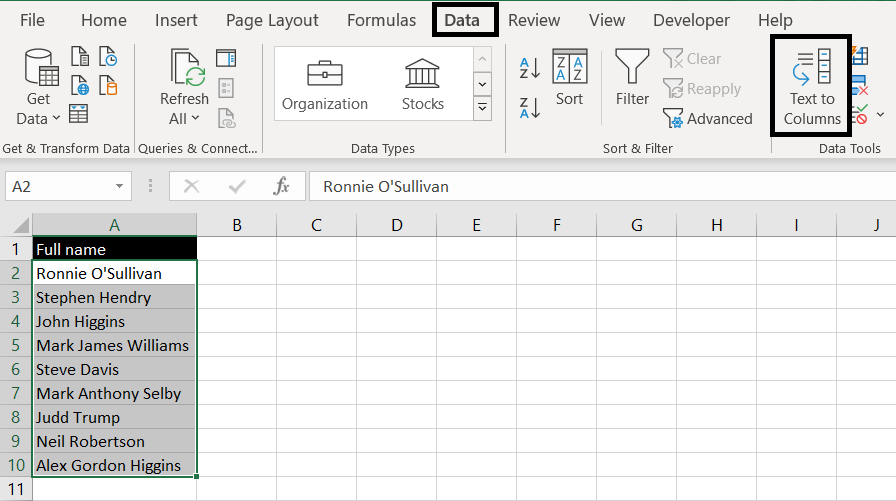3. With the Convert Text to Columns Wizard pop up, make sure “Delimited” is selected for Step 1 and click Next.
4. For Step 2, make sure Space is selected: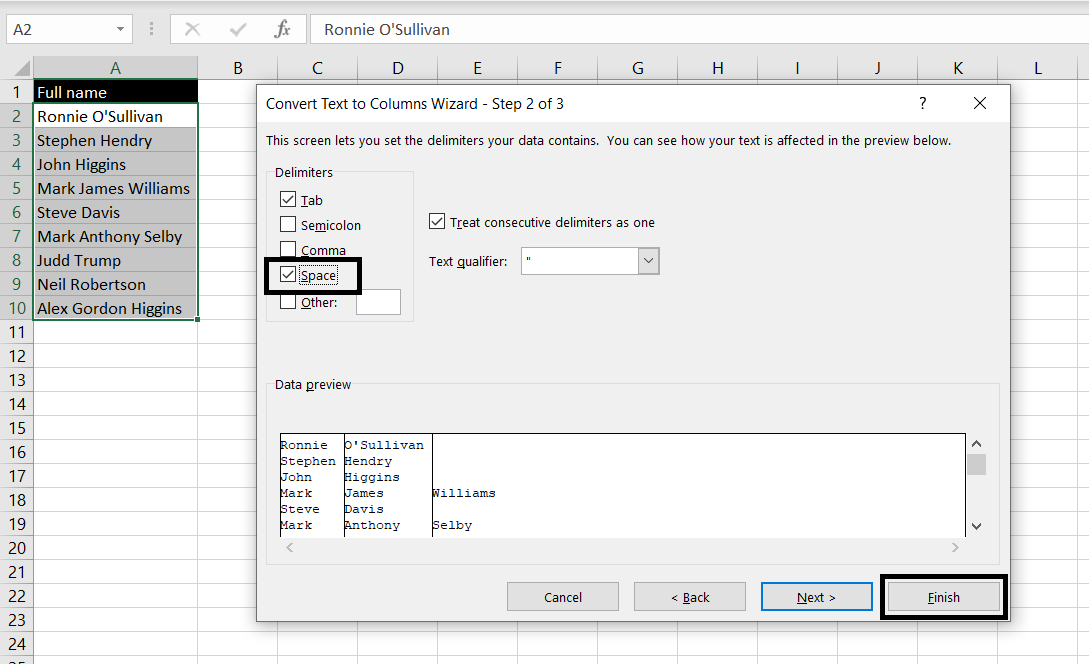5. Click Finish

And Excel will return with following result: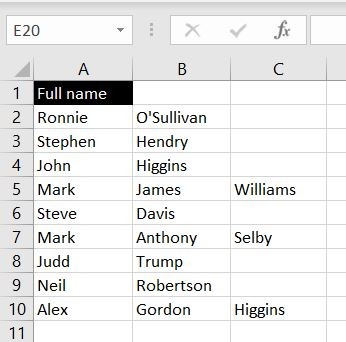Column A is the list first names. But the last name could be in Column B or C depending if the person has a middle name or not. To get a full list of the last names, we can add a formula in Column D: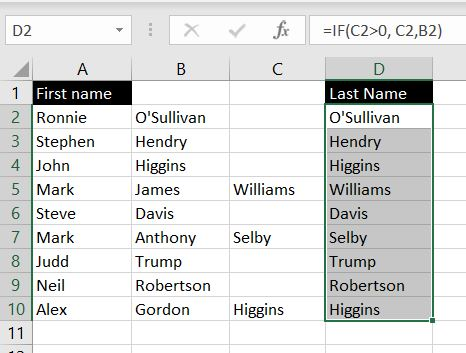=IF(C2>0, C2, B2)

It’s a simple formula. What it does is it checks if there’s a value in Column C. If Column C is blank, the value in Column B will be returned.

As a final touch, before removing data in Columns B and C:

1. Highlight the list of last names in Column D
2. Copy & Paste Values

This will remove the formulas and then we can delete Columns B and C. We will be left with: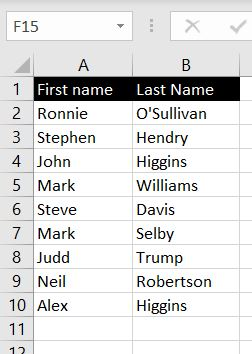## Separate Names Using Formulas

Using formulas to separate first and last names is a little more complicated. However you could also just copy and paste the formulas below.

### First Names

First names are a lot easier. We need to use the FIND and LEFT functions. First we find out where the first space is in the full name and we use the LEFT function to return all the characters to the left of it: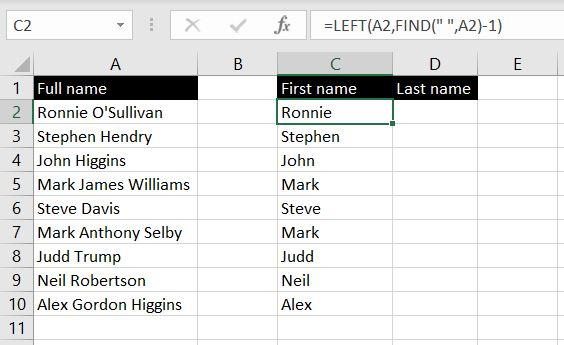=LEFT(A2, FIND(” “, A2)-1)

The reason why we need to minus one after the FIND function is because we don’t want Excel to return the space as well. To prevent that, we FIND the location of the space and use the LEFT function to return characters up to but not including the space.

Last Name

This one is a bit trickier because some names have middle names and some don’t. To do this, we need a combination of RIGHT, FIND, LEN and SUBSTITUTE functions. To break this down, there are two scenarios here: names with middle names and names without middle names

#### Names Without Middle Names

For names without middle names, it works very much like first names. Except, we will be using the RIGHT function: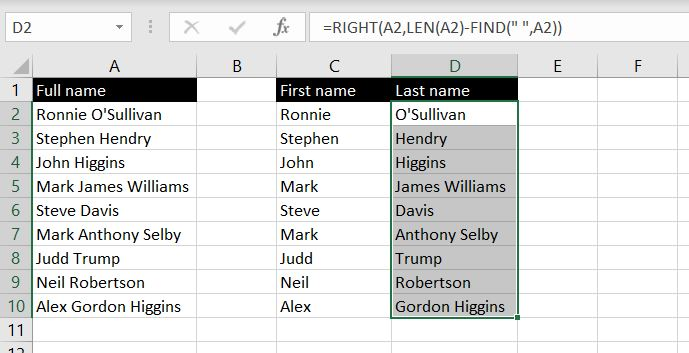=RIGHT(A2, LEN(A2)-FIND(” “, A2))

First we FIND out where the space is in the full name: FIND(” “, A2). And then we find out how many characters there are in total with the full name: LEN(A2). We then minus the two to work out how many characters there are from the space to the end of the name: LEN(A2)-FIND(” “, A2). And this is the number of characters we want the RIGHT function to return.

#### Names With Middle Names

For names with middle names, we need to find the location of the second space (the one between the middle name and the last name). To do that, we first SUBSTITUTE the first space another character and then we can use the FIND function to look for the location of the second space:

=FIND(” “, SUBSTITUTE(A3,” “, “@”, 1)

For example, the SUBTITUTE function change “Mark James Williams” to “Mark@James Williams”. The FIND function will then be able to find the location of the space between the middle name and the last name.

After we find the location of that space, the rest is the same. We use LEN to work out the total number of characters in the full name. We then subtract the location of that space from the total number of characters. This will tell us how many characters there are with the last name: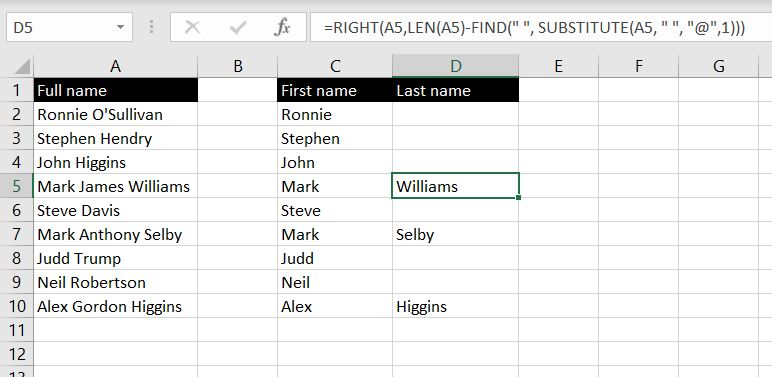=RIGHT(A5, LEN(A5)-FIND(” “, SUBSTITUTE(A5, ” “, “@”, 1)))

#### Putting Everything Together

So now we’ve worked out both scenarios, we just need to see which formula to use in each scenario. That is, to use the first formula if there is no middle name and to use the second formula if there is a middle name. And the difference between the two is the number of spaces there are in the full name. A full name with two spaces would have a middle name. And a full name with only one space would have no middle name.

We can work this out by first removing the space(s) with the SUBSTITUTE function – to swap ” ” with “”. We can then compare the number of characters before and after.

For example, with “Ronnie O’Sullivan”;

=LEN(“Ronnie O’Sullivan) will return 17

=SUBSTITUTE(“Ronnie O’Sullivan”, ” “, “”) will return “RonnieO’Sullivan”. And =LEN(“RonnieO’Sullivan”) will return 16

And the difference:

=LEN(“Ronnie O’Sullivan”)-LEN(SUBSTITUE(“Ronnie O’Sullivan”, ” “, “”) will return 1

This would tell us there is only one space in the name “Ronnie O’Sullivan”.

Now we just need to put this into an IF function. If the formula above returns a 1, we know it does not have a middle name. Otherwise, there is a middle name: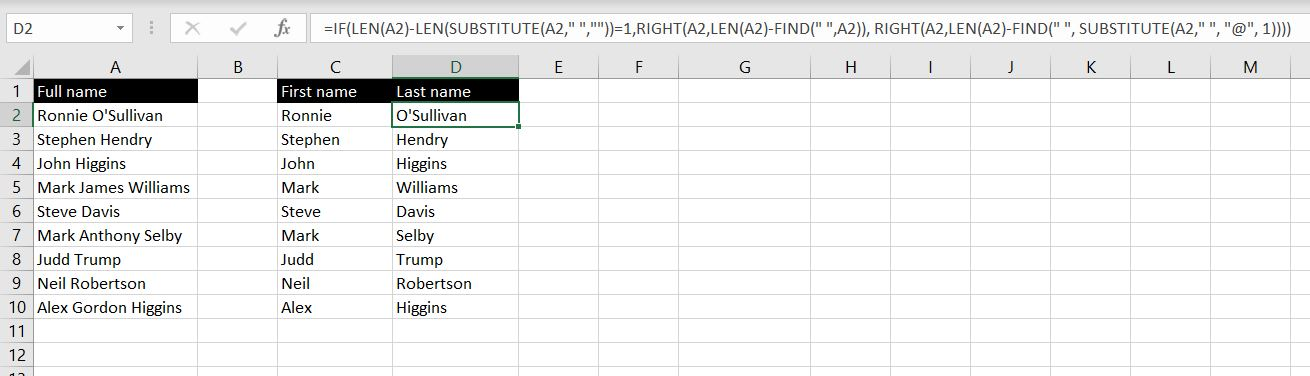=IF(LEN(A2)-LEN(SUBSTITUTE(A2,” “,””))=1,RIGHT(A2,LEN(A2)-FIND(” “,A2)), RIGHT(A2,LEN(A2)-FIND(” “, SUBSTITUTE(A2,” “, “@”, 1))))

And with this formula above, we can draw out the last name from a full name regardless there is a middle name or not.

Now we have two different ways to separate full names in Excel. One is to use Text to Columns and the other is to use formulas. Feel free to leave a comment below if you have any questions or comments on this article!